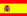You are here : > Home> Utilities > Mathematics & Statistics > Rule of three - direct proportion >

### Rule of three - direct proportion

The rule of  three is a method  to  solve  proportions. If  you have three numbers: a, b, c, such that,   ( a / b = c / x),  (i.e., a: b :: c: x ) you can calculate the unknown value (x).
Direct Proportion
If, a change in a quantity causes a change in unknown quantity, in the same proportion, the amounts are in direct proportion.
Example
If, 4kg of a product cost \$36.00, how much would the cost of 18kg?
Problem formulation.
4   kg -   cost \$36.00
18  kg -  cost x
Full text: Rule of three - direct proportion.
Enter data as required, the result is automatically displayed after you click on "Calculate"

 Rule of three - direct proportion Decimal places: a = c = b = x  =